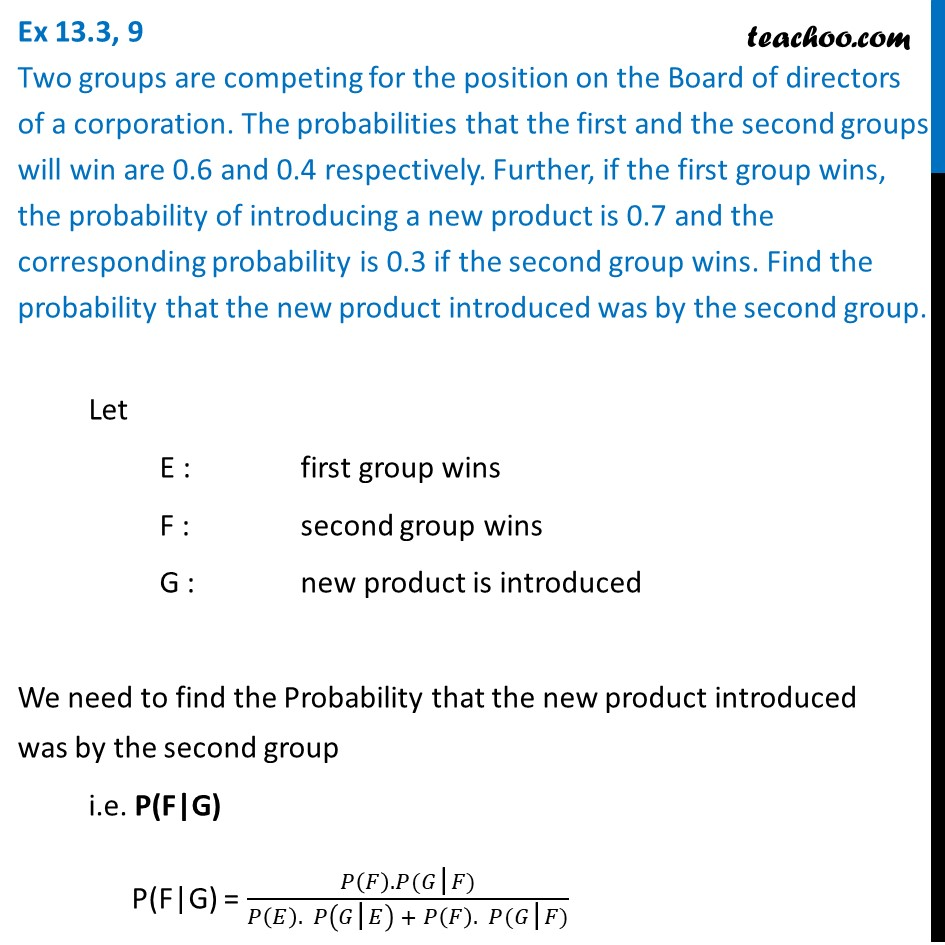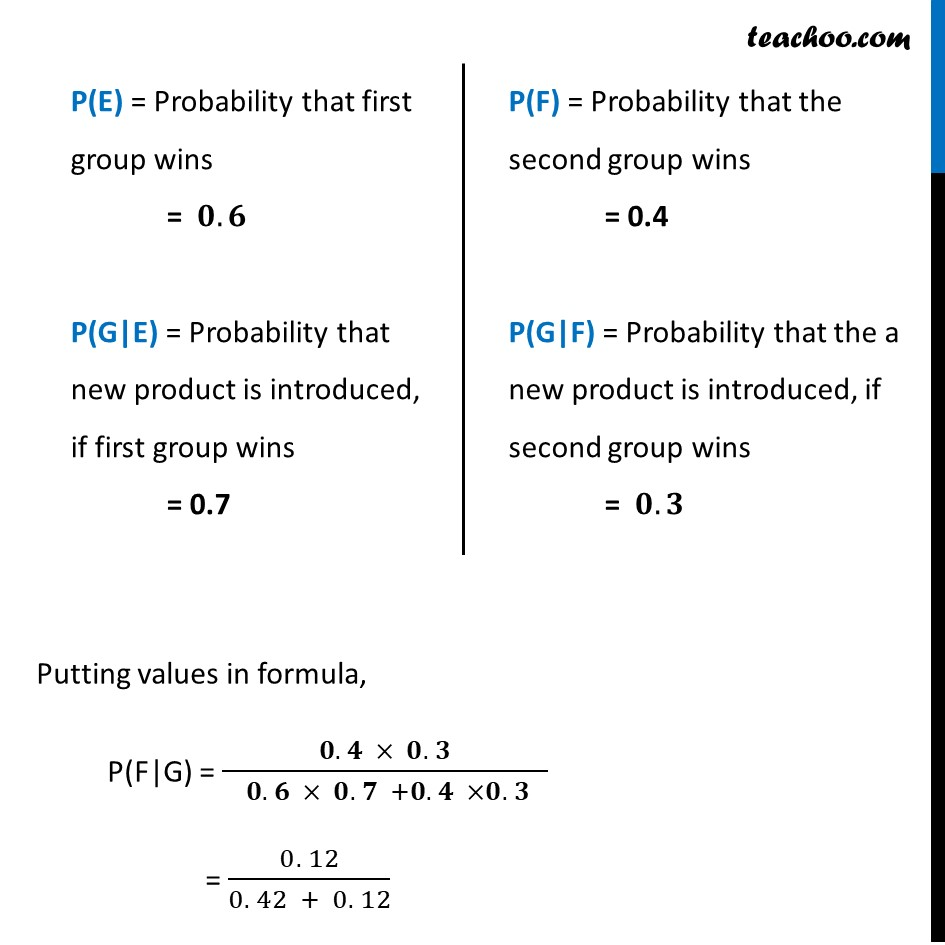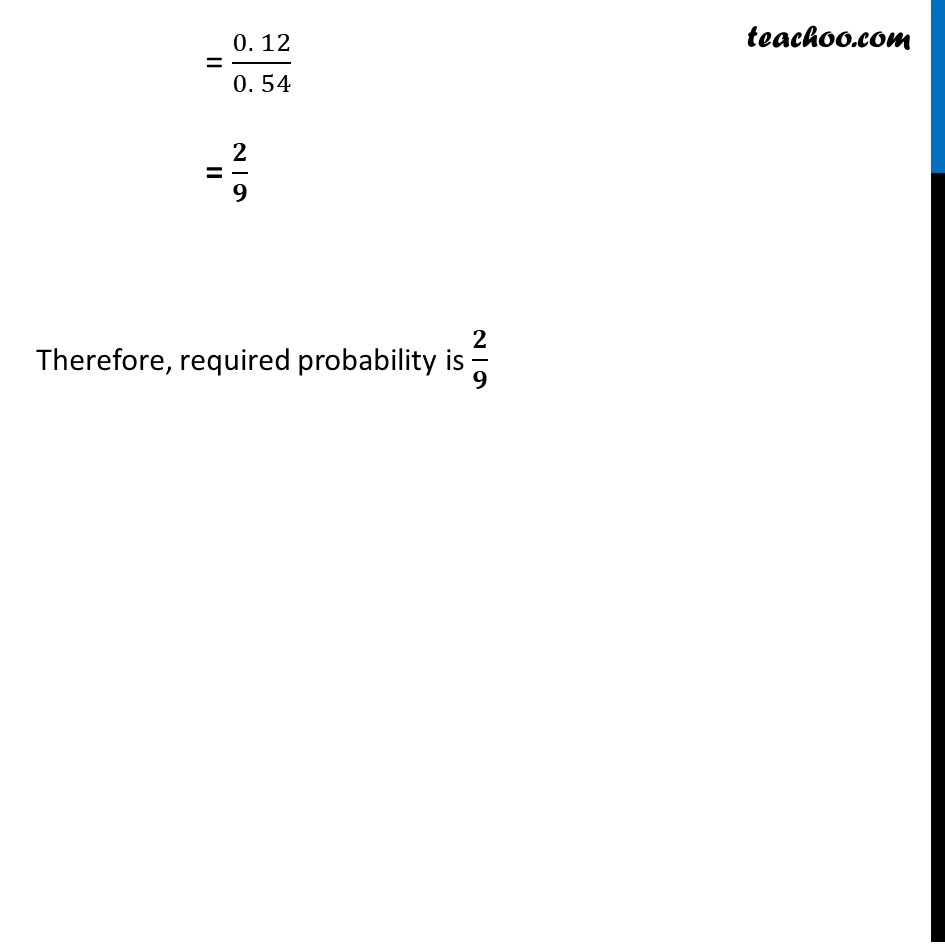Ex 13.3

Chapter 13 Class 12 Probability
Serial order wiseLearn in your speed, with individual attention - Teachoo Maths 1-on-1 Class

### Transcript

Ex 13.3, 9 Two groups are competing for the position on the Board of directors of a corporation. The probabilities that the first and the second groups will win are 0.6 and 0.4 respectively. Further, if the first group wins, the probability of introducing a new product is 0.7 and the corresponding probability is 0.3 if the second group wins. Find the probability that the new product introduced was by the second group. Let E : first group wins F : second group wins G : new product is introduced We need to find the Probability that the new product introduced was by the second group i.e. P(F|G) P(F|G) = (𝑃(𝐹). 𝑃(𝐺"|" 𝐹))/(𝑃(𝐸). 𝑃(𝐺"|" 𝐸) + 𝑃(𝐹). 𝑃(𝐺"|" 𝐹)) "P(E)" = Probability that first group wins = 𝟎.𝟔 "P(G|E)" = Probability that new product is introduced, if first group wins = 0.7 "P(F)" = Probability that the second group wins = 0.4 "P(G|F)" = Probability that the a new product is introduced, if second group wins = 𝟎.𝟑 Putting values in formula, "P(F|G)" = (𝟎. 𝟒 × 𝟎. 𝟑)/( 𝟎. 𝟔 × 𝟎. 𝟕 +𝟎. 𝟒 ×𝟎. 𝟑 ) = (0. 12)/(0. 42 + 0. 12) = (0. 12)/(0. 54) = 𝟐/𝟗 Therefore, required probability is 𝟐/𝟗